# One‑Way ANOVA in SPSS# One Way ANOVA in SPSS

One Way Analysis of Variance (ANOVA) is used to determine whether there are any statistically significant differences among the means of three or more independent groups.

One Way ANOVA has the following hypothesis.

Null hypothesis: µ1 = µ2 = µ3  …µk (k is the number of independent comparison groups)

Alternative hypothesis: All means are not equal.

Assumptions

1. Dependent variable should be continuous.

2. Independent variables should be of more than two categories.

3. The dependent variable should be approximately normally distributed for each category of the independent variables.

4. Homogeneity of variances is required, i.e., variances of the dependent variable should be equal.

5. Significant outliers should not be present.

Here, we have taken ‘Depression Score’ as the dependent variable and ‘Body mass index’ as the independent variable. We labelled 1 as ‘Healthy weight’, 2 as ‘Underweight’, 3 as ‘Overweight’ and 4 as ‘Obesity’.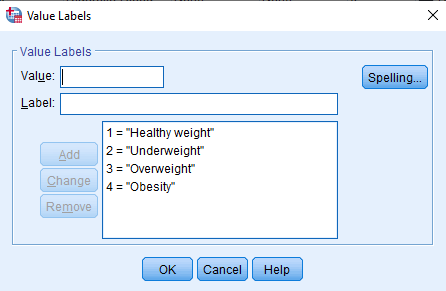In SPSS, One Way ANOVA is found in Analyze > Compare Means > One Way ANOVA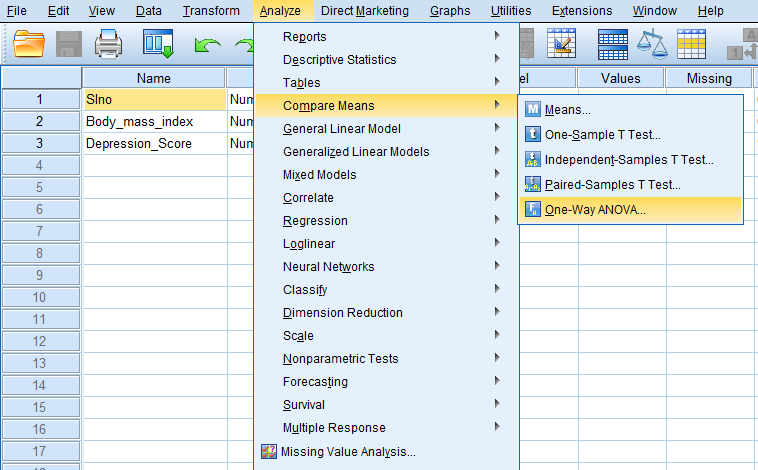Then, we will get ‘One Way ANOVA’ dialog box.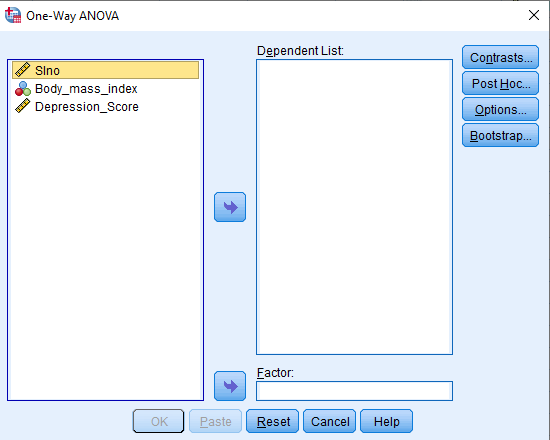We have to add dependent variable (Depression Score) in the ‘Dependent List’ box and add independent variable (Body mass index) in the ‘Factor’ box.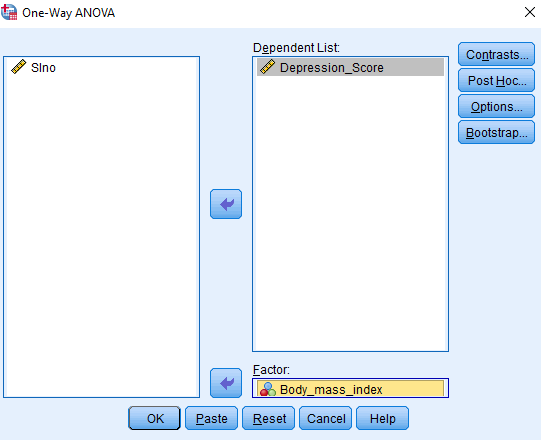In the ‘Post Hoc Multiple Comparisons’ dialog box, we have to add ‘Tukey’ and click on ‘Continue’.In the ‘Options’ dialog box, add ‘Descriptive’ in the ‘Statistics’ group.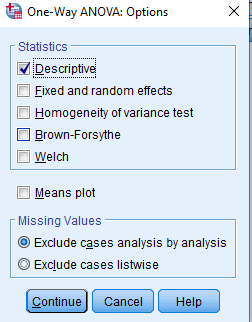Click on ‘Continue’ and ‘Ok’.

Output of One Way ANOVA
First, we will get the ‘Descriptives’ table. It provides descriptive statistics for each of the groups (Healthy weight, Underweight, Overweight, and Obesity) of the dependent variable.Then, we will get the ‘ANOVA’ table, wherein the analysis is found.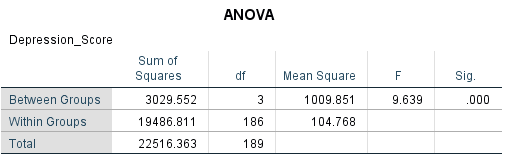Column ‘F’ gives the F Statistic and Column ‘Sig.’ gives the p value that corresponds to the F statistic (9.639) with df numerator (3) and df denominator (27). Here, p value is 0.000 and it is less than 0.05. Therefore, we reject the null hypothesis and conclude that at least one of the groups’ means is different from the rest.

Then, we will get the ‘Multiple Comparisons’ table, which gives information about the difference of means of various groups.‘Multiple Comparisons’ table gives Tukey post hoc multiple comparisons among each of the four groups. The table demonstrates a statistical significant difference between the depression scores of underweight and healthy weight people (p = 0.000), underweight and obesity people (p = 0.000), and overweight and underweight people (p = 0.43).

Data: One_way_ANOVA_data.sav

“Rehoboth Academic Services” is a premium institute supporting PhD & Master’s Thesis since 2013. We offer editingproofreadingpaper preparationstatistical analysis, formatting and plagiarism checking services. We have helped more than 1000+ research scholars in most of the subjects and universities across the globe in the last seven years. We also conduct workshops on Art of Thesis Writing, Academic Integrity, Research Paper writing, Systematic Review writing, Increasing citation score, SPSS Foundation & Advanced, Jamovi, JASP, SmartPLS and SEM workshops. If you need our assistance please call + 91 9731988227, +91 9741871657.

Are you interested to attend our Workshops?